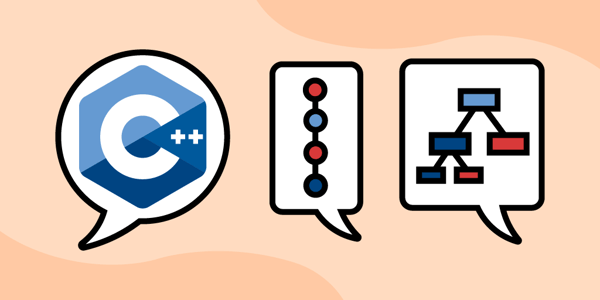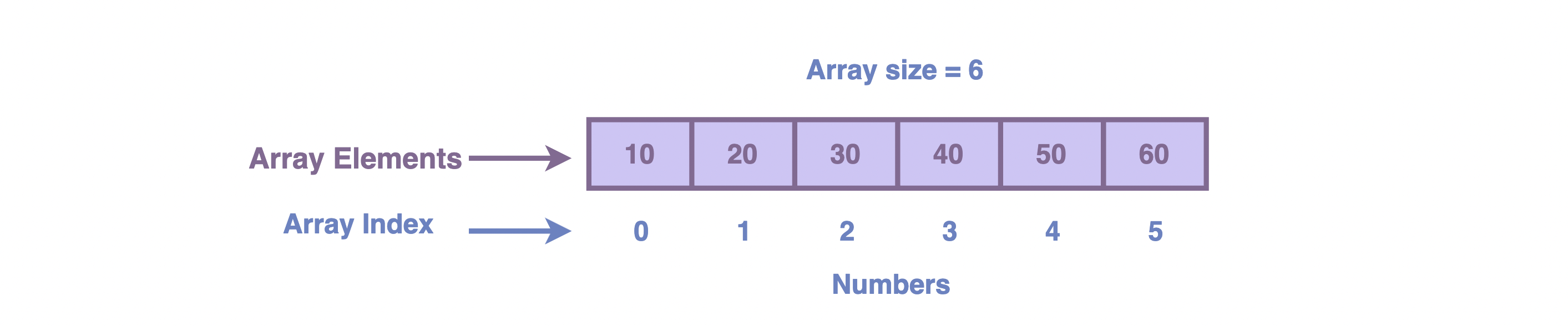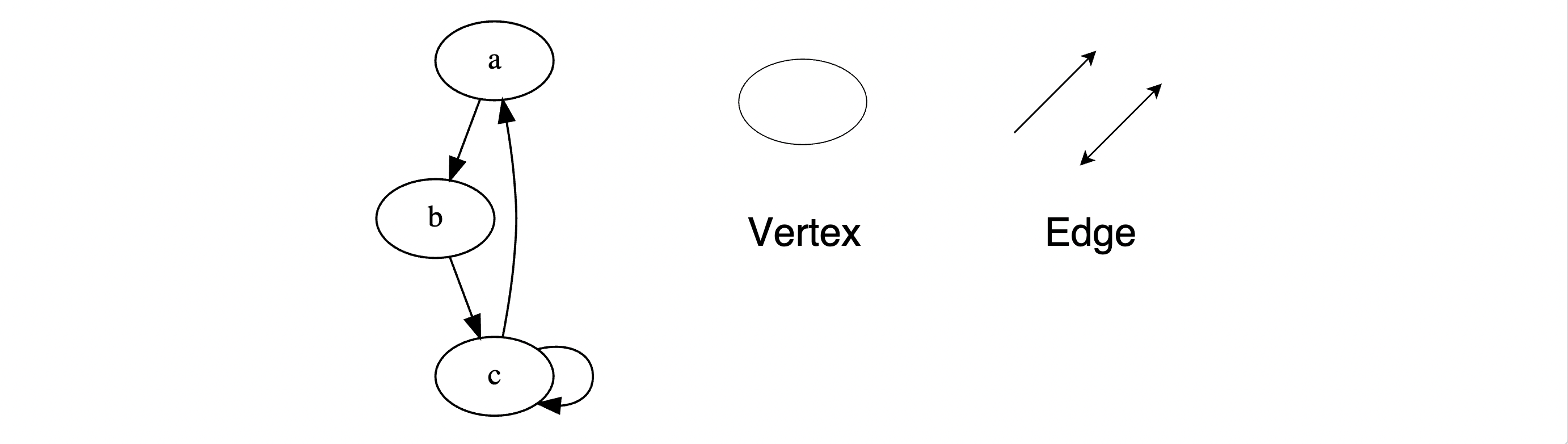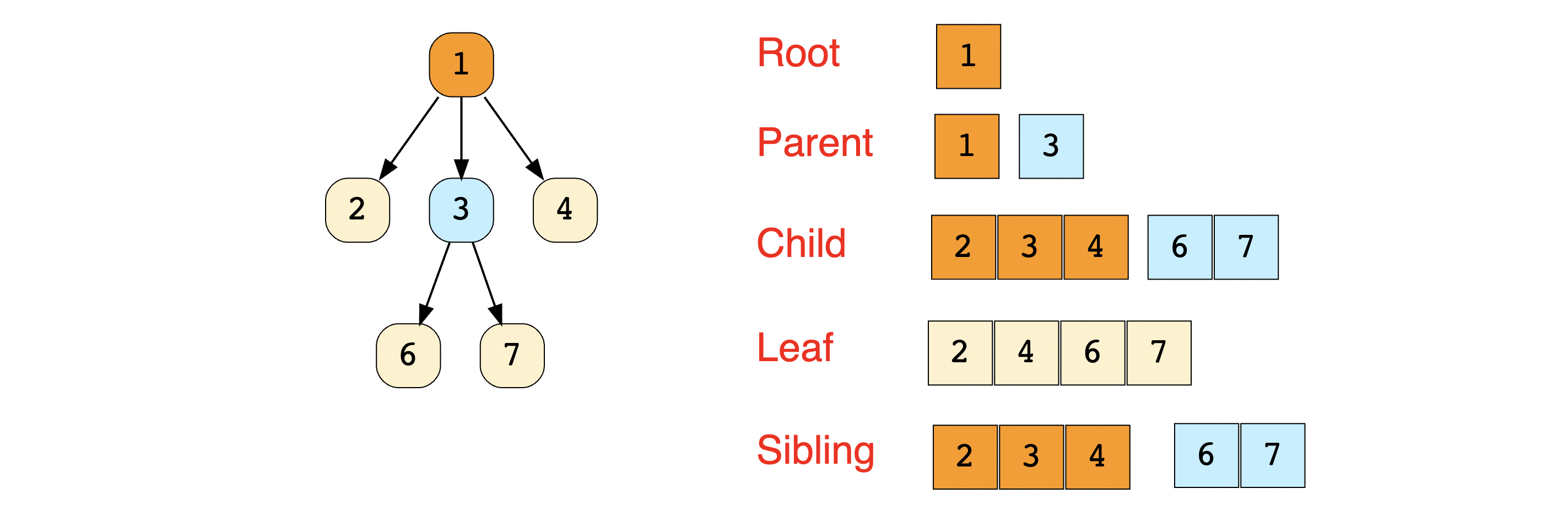Home/Blog/Interview Prep/9 C++ data structures you need to know for your coding interview

# 9 C++ data structures you need to know for your coding interview

Dec 15, 2021 - 19 min readErica VartanianData structures are formats used to organize, store, and modify data. Data structures are a fundamental component of computer science and software engineering. They can be implemented in any programming language, including the C++ programming language. To secure your dream job, it is crucial to understand how to select the most appropriate data structure for solving specific problems and creating efficient algorithms. To do so, you should consider the advantages and disadvantages of each data structure.

Today, we’ll cover 9 data structures using C++ that you need to know for your coding interview.

We’ll cover:

## What are C++ data structures?

Data structures are formats used to organize, store, and manage data. You can use data structures to access and modify data efficiently. There are various types of data structures. The type you use will depend on the application you’re using. Each data structure has its advantages and disadvantages.

Data structures generally fall under these two categories:

• Linear data structures: Elements are arranged sequentially (e.g. arrays, linked lists, stacks, queues)
• Non-linear data structures: Elements are not arranged sequentially, but are stored within different levels (e.g. trees, graphs)

## 9 C++ data structures you need to know for your interview

### 1. Arrays

An array is a sequential list of values. You can use arrays to store and access multiple values of the same data type under one identifier. Arrays makes it easier to perform operations on an entire dataset. They’re also great for retrieving data.

There are one-dimensional arrays and multi-dimensional arrays. The values stored in an array are called elements. Elements are stored in consecutive blocks of memory called indexes. The size of an array is determined by the number of elements it contains. Pictured is a one-dimensional array with a size of six.The general syntax for initializing a one-dimensional array is:

ArrayName [ArrayIndex] = value;

In this code, we initialize an array named Example that stores 200 at index 0 and 201 at index 1.

#include <iostream>using namespace std;int main() {  int Example;  Example = 200;  Example = 201;}

For your C++ interview preparation, check out this tutorial on passing arrays to functions in C++.

### Implementation of arrays

As we saw earlier, we implement arrays in C++ as fixed-size blocks of contiguous memory. The size of the array is determined when it is created and cannot be altered later on. Each element in the array is stored in a contiguous block of memory and can be accessed using an index, which is an integer that specifies the position of the element in the array.

Here is a code example of how to declare and initialize an array of integers in C++:

int arr; // Declare an array of 10 integers// Initialize the elements of the arrayarr = 1;arr = 2;arr = 3;// ...arr = 10;

We can also employ an initializer list to initialize the elements of the array when it is declared:

int arr = {1, 2, 3, 4, 5, 6, 7, 8, 9, 10};

To access an element in the array, you can use the index operator ([]). For example, to access the first element of the array, we can use the expression arr .

It’s important to note that in C++, arrays are zero-indexed, which means that the index of the first element is 0 by default, and the last element’s index is the size of the array minus 1.

Here is an example of how to iterate through the elements of an array and print them to the console:

for (int i = 0; i < 10; i++){    std::cout << arr[i] << std::endl;}}

Let’s combine the initialization and printing of an array:

#include <iostream>using namespace std;int main(){   int arr = {1, 2, 3, 4, 5, 6, 7, 8, 9, 10};   for (int i = 0; i < 10; i++)   {       std::cout << arr[i] << std::endl;   }}

### 2. Stacks

. Stacks are containers of objects based on arrays that follow a Last-In-First-Out (LIFO) order, meaning that the most recently added item is removed first. Stacks are often used with the recursive backtracking algorithm and as the mechanism supporting the “undo” or “back” button in applications and browsers. Compilers often use stacks to parse the syntax of expressions before translating them into low-level code.

With stacks, we only remove the most recently added item. A popular example is a stack of lunch trays in a cafeteria.

### Implementation of stacks

Stacks can be implemented using various data structures, including:

• Arrays: As defined earlier, arrays are sequential collections of elements stored in contiguous memory locations. Arrays are easy to implement and offer fast access to the elements, but they have a fixed size and cannot be altered once they are created.

• Linked lists: Linked lists are collections of elements linked together using pointers. Linked lists are more flexible than arrays because they can grow and shrink when required, but the downside is that they are more complex to implement and require extra memory to store pointers.

• Dynamic arrays: These are arrays that can automatically resize themselves when required. Dynamic arrays combine the simplicity of arrays with the flexibility of linked lists, but are generally slower than other data structures due to having to copy elements when an array is resized.

• Vector: A vector is a dynamic array with an added ability to shrink. It allows for efficient insertion and deletion of elements at the end of the stack, but may be slower for inserting or deleting at the beginning or middle of the stack.

• Deque (Double-ended queue): A deque is a dynamic array that allows for efficient insertion and deletion of elements at both the beginning and end of the stack. It offers the fastest insertion and deletion among the stack implementations, but may be slower for accessing the middle elements and therefore should be used sparingly.

### 3. Queues

Queues are containers of objects based on arrays that follow a First-In-First-Out (FIFO) order, meaning that the least recently added item is removed first. Queues are helpful in cases where the FIFO order of data needs to be maintained, such as for caching and handling real-time system interruptions. A real-life example of a queue data structure is a line at a ticket counter or amusement park ride, where the people at the front of the line are the first to be served.

### Implementation of queues

Queues can be implemented using various data structures, including:

• Arrays: these are are sequential collections of elements stored in contiguous memory locations and are easy to implement, but they have a fixed size and cannot be altered once they are created.

• Linked lists: These are collections of elements linked together using pointers and are more flexible than arrays, but they are more complex to implement and require extra memory to store pointers.

• Dynamic arrays: These are arrays can automatically resize themselves when required and combine the simplicity of arrays with the flexibility of linked lists, but they are generally slower than other data structures.

• Heaps: They are complete binary trees where the parent node is either greater than or equal to (in the case of a max heap) or less than or equal to (in the case of a min heap) its children and are more complex to implement, but they can be more efficient for certain operations, such as finding the maximum or minimum element in the queue.

Linked lists are linear data structures consisting of a collection of nodes that represent a sequence. Each node contains a value and a pointer to the next node in the sequence. Linked lists are great for adding and deleting nodes. However, they’re not great for retrieving nodes, since each node is only aware of the node next to it.

There are both singly linked lists and doubly linked lists. A singly linked list is the most basic form of a linked list, where each node is only aware of the next node in the sequence. In a doubly linked list, each node points to the node preceding and following it in the sequence. The direction of these links are often distinguished as “forward” and “backwards,” or “next” and “prev(ious)”.

Be sure you know how to reverse a linked list before going to a C++ interview.

Some common approaches to implementing a linked list include:

• Singly-linked list: In this approach, each node in the list has a pointer to the next node, but not to the previous node. This allows faster insertion and deletion of elements but does not allow efficient traversal of the list in reverse order.

• Doubly linked list: Via this technique, each node has a pointer to the next and previous nodes. This ensures faster insertion and deletion of elements and efficient list traversal in both forward and reverse order.

• Circular linked list: Here, the end node in the list points back to the beginning node, forming a circular structure. This approach enables efficient traversal of the list, but requires additional logic to determine when the end of the list has been reached.

• Using a sentinel/dummy node: In this method, we implement the list as a singly linked list but with an additional “sentinel or dummy” node at the start and/or end. The sentinel node simplifies the logic for inserting and deleting elements, as it always exists and does not need to be checked for null pointers.

### 5. Hash tables

Hash tables store key and data pairs. They’re usually implemented using arrays, where each element has its own unique index value to enable quick access. While you could assign the key (an integer value) as the index for data, this gets complicated in the case of large keys. Instead, the hashing technique is used to generate indexes for the elements.

This figure represents key and data pairs stored in a hash table.The hashing technique uses a hash function to convert large keys into smaller keys which can then be stored in a hash table. A good hash function should meet the following conditions:

• Easy to compute
• Distributes data uniformly (without clustering)
• Minimizes collision

A hash table’s performance depends on the size of the hash table, the hash function used, and the collision handling method.

Collision is something we try to avoid when hashing. Collision refers to instances where two different keys passed to a hash function return the same index. The chances of collision increase when we’re dealing with large keys. Collision handling methods help to work around collisions, and include the following common strategies: linear probing, chaining, and resizing the array.

Hash tables are excellent data structures for algorithms prioritizing search operations. Insertion and search operations are quite fast in hash tables. That is, in the absence of collision these operations take constant time $O(1)$. Hash tables are a popular topic for C++ coding interviews.

### Implementation of hash tables

We can implement a hash table in C++ in several different ways such as:

• Using an array and a hash function: We can use an array as the underlying data structure, and a hash function to map keys to indices in the array. The hash function will take a key as the input and will return an index in the array where the key-value pair should be stored. Collisions can be managed via a separate chaining technique, where each index in the array stores a list of key-value pairs that hash to that index.

• std::unordered_map: C++ provides a built-in unordered associative container, std::unordered_map, that implements a hash table. It stores key-value pairs and uses a hash function to map keys to indices in an underlying array. The std::unordered_map class is part of the C++ Standard Template Library (STL) and is implemented using a hash table with separate chaining. It comes with an average time complexity of O(1) for most operations.

• std::map: Another option is to use a std::map, which is a built-in ordered associative container in the STL. A std::map stores key-value pairs in a balanced binary search tree, and has an average time complexity of O(log n) for most operations. While a std::map is not technically a hash table, it can be used to implement similar functionality.

• boost::unordered_map: With a Boost library, you can use a boost::unordered_map, which is similar to std::unordered_map but with additional features and functionality.

### 6. Graphs

Graphs are non-linear data structures. They consist of a finite set of vertices (i.e. nodes) connected by a set of edges. The order of a graph refers to the number of vertices in the graph. A graph’s size corresponds to its number of edges.This illustration represents vertices and edges. The graph also represents a cyclic graph. A graph is cyclic if at least one node has a path that directs back to itself.

Graphs are frequently used to model real-life relationships in various contexts ranging from social networks to neural networks.

There are three common types of graphs:

• Undirected graphs: A graph in which all edges are bi-directional
• Directed graphs: A graph in which all edges are uni-directional
• Weighted graphs: A graph in which all edges are assigned a value, called a weight

You’ll likely be asked to traverse a graph in your interview. For your C++ interview preparation, make sure you’re comfortable with using common graph algorithms such as breadth-first search and depth-first search.

### Implementation of graphs

There are quite a few ways to implement a graph in C++.

• Adjacency matrix: This is just a two-dimensional array that represents the connections between vertices in the graph. For instance, if there is an edge between vertex i and vertex j, then the adjacency matrix at row i and column j would be set to 1 (or true). If there is no edge between the vertices, then the value would be set to 0 (or false).

• Adjacency list: Another way to implement a graph in C++ is to use an adjacency list, which is a vector (or list) of linked lists. Each element in the vector represents a vertex in the graph, and the linked list stores the edges connecting to that vertex.

## Trees (#7, 8, and 9)

Next, we’ll cover binary trees, binary search trees, and tries. Before we do, let’s learn about the category they all belong to: trees.

Trees are abstract data types that model a hierarchical tree structure. Trees consist of nodes (i.e. vertices) and edges that connect them. Linked nodes are called “parent” and "children” nodes. Unlike graphs, a cycle can’t exist in a tree. In a tree, there is no more than a single path connecting any two nodes.

Some basic terminology for trees are:

• Root node: A node with no parent nodes
• Parent nodes: A node connected to one or more child nodes
• Child node: A node that is connected to an upper node (parent node)
• Sibling node: Nodes that share the same parent node
• Leaf node: A node with no child nodes
• Level: Each step of the tree, beginning from level 0 at the root node and increasing incrementally from top to bottom
• Subtree: A portion of the tree that forms a complete tree on its own

This image illustrates the relationships in a tree.### 7. Binary trees

Binary trees are one type of tree data structure. You have a binary tree whenever you have a tree wherein each node has no more than two children. Each parent node’s children are referred to as the left child and right child.

Binary trees consist of the following types:

• Complete binary tree: A binary tree in which all levels of the tree are filled, with the exception of the last level, which can be filled from left to right (refer to illustration)• Full binary tree: A binary tree for which each node has zero or two children

• Perfect binary tree: A binary tree that is both full and complete (i.e. all interior nodes have two children and all leaves are at the same level)

### 8. Binary search trees

Binary search trees allow binary search algorithms to quickly lookup, add and remove data elements. Binary search is a search algorithm that finds the position of a target value within a sorted array. The algorithm does this by comparing the target value to the middle element of the array.

A binary tree is a binary search tree if it satisfies the following conditions:

• Values of all nodes in the left sub-tree are equal to or less than the value of the current node
• Values of all nodes in the right sub-tree are equal to or greater than the value of the current node
• The above conditions apply to both left and right sub-trees

This illustration represents a binary search tree.Binary search trees are often used in search applications where data is continuously entering and leaving. Operations such as iterative and recursive searching and traversal can be applied to binary search trees. Binary search trees will likely be involved in solutions to C++ coding interview questions.

### 9. Tries

A trie is another type of search tree. Tries are efficient data structures for solving programming problems involving strings. They’re also known as a prefix tree because they’re based on the prefix of a string.

The term “trie” is derived from the word “retrieve” because tries are great for retrieving data.

Tries consist of a combination of nodes, wherein each node represents a unique letter of the alphabet. A trie consists of one empty root node which links to other nodes. A trie can’t have duplicate nodes.

Each node in a trie contains:

• A value (can be null)
• An array of references to child nodes (can also be null)

Tries were introduced to help efficiently represent sets of words. They are frequently used for autocomplete and search functionalities, as well as for IP routing.

### Implementation of trees

We can implement trees in C++ in several ways such as by using:

Node-based data structure: This involves creating a TreeNode struct that holds a value and pointers to its children, and using these nodes to build the tree. This approach is flexible and widely used and allows you to easily add, remove, and modify nodes in the tree.

Array-based implementation: We can also use an array-based representation of the tree, where each node is represented by an index in the array. This approach can be more memory-efficient than a node-based implementation, since it does not require additional memory for pointers. However, it may be more difficult to insert and delete nodes from the tree, because it requires shifting elements in the array.

Adjacency matrix: An adjacency matrix is basically a 2D matrix that represents the edges between nodes in a graph. In the case of a tree, the adjacency matrix is symmetric, with a 1 in the (i, j) position depicting that there is an edge between nodes i and j,and a0` indicating no edge. This approach can be efficient for trees with a small number of nodes and a large number of edges, but can be inefficient for trees with a large number of nodes and a small number of edges.

Container class: We can also implement a tree data structure by creating a container class that holds the root node and provides methods for manipulating the tree. A container class is simply a class that is used to store and manage a collection of objects. In the case of a tree, the container class could store the nodes of the tree and provide methods for inserting, deleting, and traversing the tree. This can be a convenient way to encapsulate the tree data structure and provide a user-friendly interface for working with

### Common and not-so-common data structures in C++

Some learners ask, “what are common and uncommon data structures in C++?” While a fair question to be asked by curious minds, the question in itself is a little odd.

In fact, it can be thought of as similar to asking the question, “What are common and uncommon colors used in painting?”

The answer is: it depends on what you’re trying to do.

The data structures we use are always dependent on what we’re trying to solve, so the question would be better phrased as “What are more common vs less common problems that we try to solve with C++?”

Generally speaking, more common data structures in C++ are:

• Arrays
• Vectors
• Trees
• Maps
• Sets

Here are some less common data structures in C++:

• Hash tables
• Tries
• Heaps
• Graphs

In conclusion, it is important to consider the specific problem we are trying to solve when choosing which data structures to use in our C++ code. Asking “What are more common vs less common problems that we try to solve with C++?” can help guide our decision-making process and ensure that we are using the most appropriate data structures for the task at hand.

## Start mastering the C++ interview today

Demonstrating your ability to work with data structures will be essential to acing your coding interview. During your C++ interview preparation, be sure you have a strong understanding of how to leverage the data structures in this article.

To help you master C++ data structures, check out our course Grokking Coding Interview Patterns in C++. This interactive learning path consists of several modules and quizzes to help programmers like you walk into your interview with confidence.

### Continue learning about data structures and C++WRITTEN BYErica Vartanian

Join a community of 1.7 million readers. Enjoy a FREE, weekly newsletter rounding up Educative's most popular learning resources, coding tips, and career advice.

Learn in-demand tech skills in half the time